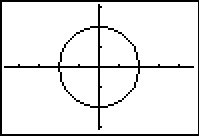Conics:  Graphing Circles

 A.  center at the origin: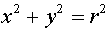B.  center at (h, k):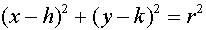Example A:
Graph: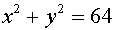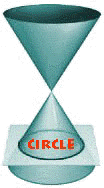Solution:  Enter the equation into Y= by solving for y.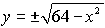Enter the positive square root into Y1. Enter the negative square root into Y2, or enter the negation of Y1. If you choose ZOOM #6 (the standard window), the graph will appear to be an ellipse rather than a circle due to the 3/2 aspect ratio of the viewing screen (the standard viewing screen is not a square). Choose ZOOM#5 ZSquare to create a viewing window where the units on both axes are the same length.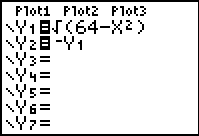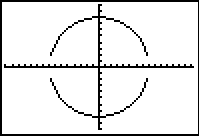NOTE:  * You may notice that the "vertical" edges of the graph may not appear in the viewing window.  The viewing screen cannot graph points that fall between pixels. * You may notice that the TRACE function will not move automatically between the two sections of the graph because the positive and negative square roots were graphed as two separate equations.  The up arrow can be used to move between the two sections of the graph.  Also, the cursor will disappear if it is moved beyond the domain for which x is defined.

Example B:  Graph: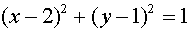Solution:  Enter the equation into Y= by solving for y.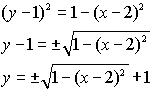Enter the equation with the positive square root into Y1. Enter the equation with the negative square root into Y2.  You cannot simply negate Y1 to obtain Y2 in this problem. Choose ZOOM#5 ZSquare to create a viewing window where the units on both axes are the same length.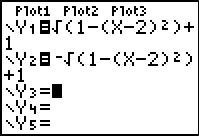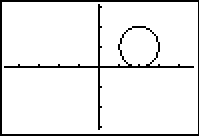Solution Using DRAW: (please follow steps in order) Press ZOOM#4 (ZDecimal) to go to a graphing screen. To graph the circle, press 2nd PRGM (DRAW) #9 Circle. Move the cursor to the "h" value of +2 by using the arrows. Move the cursor to the "k" value of +1 by using the arrows. Press ENTER to set the point for the center of the circle. Move the cursor the length of the radius (1) away from the center.  Keep track of the values at the bottom of the window. When you hit ENTER, the circle will be automatically drawn. You may need a larger window if your radius is large. (Zoom Out)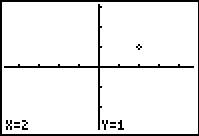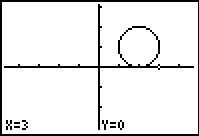Tidbit: You can use a "list" approach to deal with the plus and minus square roots:  Graph: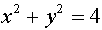which becomes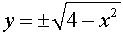Enter: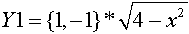Tracing still requires arrowing up and down between entries.